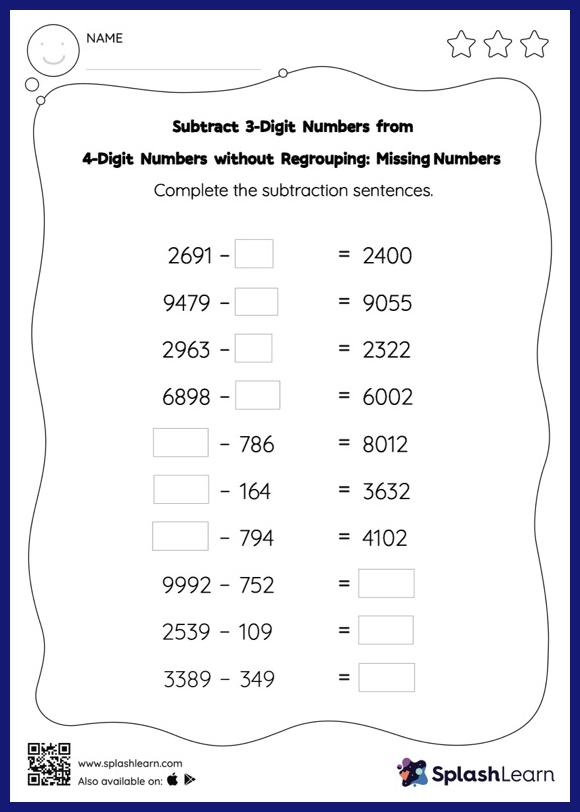# Subtract 3-Digit Numbers from 4-Digit Numbers without Regrouping: Missing Numbers Worksheet

Home > Subtract 3-Digit Numbers from 4-Digit Numbers without Regrouping: Missing NumbersThis worksheet challenges young mathematicians to hone their math skills by solving a set of problems on subtracting 3-digit numbers from 4-digit numbers without regrouping. To find the missing number on this worksheet, students can utilize the count back strategy or the connection between addition and subtraction. In subtract 3-digit numbers from 4-digit numbers without regrouping worksheet, they do not need to regroup numbers to reach the solution. In each problem, the numbers are laid out in the horizontal format. Students should try to use different strategies involving composing and decomposing numbers to solve these problems. This will help them develop flexibility and fluency.